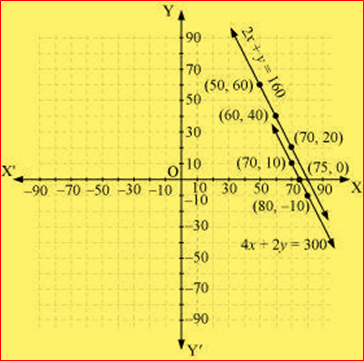# NCERT solutions for class 10 maths : Exercise Solutions

### Chapter 03-Pair of Linear Equations in Two Variables-Exercise Solutions

NCERT Book Page No 44: Exercise 3.1
Q.1. Aftab tells his daughter, “Seven years ago, I was seven times as old as you were then. Also, three years from now, I shall be three times as old as you will be.” (Isn’t this interesting?) Represent this situation algebraically and graphically.
Answer: Let the present age of Aftab be x.
And, present age of his daughter = y
Seven years ago,
Age of Aftab = x − 7
Age of his daughter = y − 7
According to the question,
(x-7) = 7(y-7)
x-7 = 7y-49
x-7y= -42 ————————-(1)
Three years hence,
Age of Aftab = x + 3
Age of his daughter = y + 3
According to the question,
(x+3)=3(y+3)
x+3 = 3(y +3)
x-3y =6 —————-(2)
Therefore, the algebraic representation is
x-7y= -42
x-3y=6
For x-7y = -42,
X = -42 + 7y
The solution table is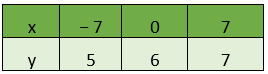For x -3y =6 ,
X = 6 +3y
The solution table is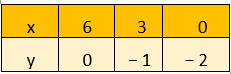The graphical representation is as follows.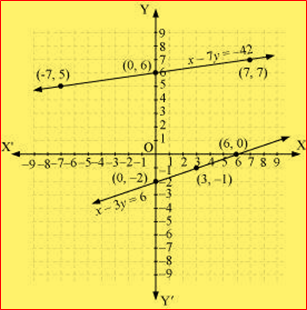Q.2. The coach of a cricket team buys 3 bats and 6 balls for Rs 3900. Later, she buys another bat and 2 more balls of the same kind for Rs 1300. Represent this situation algebraically and geometrically.
Solution: Let cost of one bat = Rs x
Cost of one ball = Rs y
3 bats and 6 balls for Rs 3900 So that
3 x + 6y = 3900 ———————(1)
Divide complete equation by 3 we get
x + 2y = 1300
Subtract 2y both side we get
x = 1300 – 2y
Plug y = – 1300, 0 and 1300 we get
x = 1300 – 2 ( – 1300 ) = 1300 + 2600 = 3900
x= 1300 -2(0) = 1300 -0 = 1300
x= 1300 – 2(1300) = 1300 – 2600 = – 1300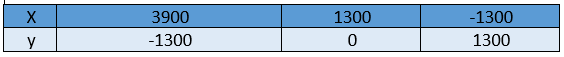Given that she buys another bat and 2 more balls of the same kind for Rs 1300
So we get
x + 2 y = 1300 ———————(2)
Subtract 2y both side we get
x = 1300 – 2y
Plug y = – 1300, 0 and 1300 we get
x = 1300 – 2 ( – 1300 ) = 1300 + 2600 = 3900
X = 1300 – 2 ( 0 ) = 1300 – 0 = 1300
X = 1300 – 2(1300) = 1300 – 2600 = -1300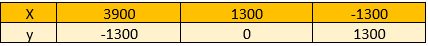Algebraic representation
3 x + 6 y = 3900 ———————(1)
x + 2 y = 1300 ———————(2)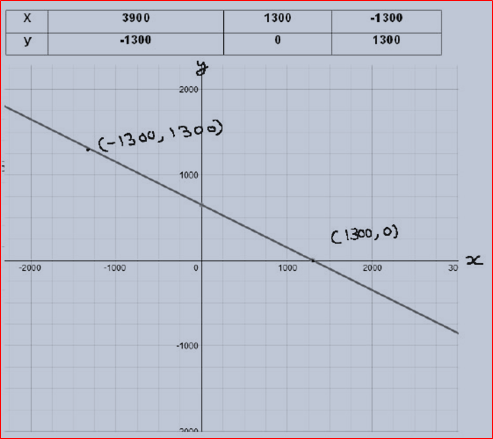Q.3. The cost of 2 kg of apples and 1 kg of grapes on a day was found to be Rs 160. After a month, the cost of 4 kg of apples and 2 kg of grapes is Rs 300. Represent the situation algebraically and geometrically.
Answer: Let the cost of 1 kg of apples be Rs x.
And, cost of 1 kg of grapes = Rs y
According to the question, the algebraic representation is
2x + y =160
4x +2y = 300
For 2x +y =160,
y= 160- 2x
The solution table is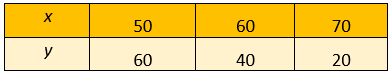For 4x + 2y = 300,
$y= \frac{300-4x}{2}$
The solution table is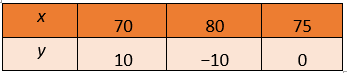The graphical representation is as follows.